﻿ 最优控制法对斜拉桥索力的控制机制

# 最优控制法对斜拉桥索力的控制机制Optimal Control Method for the Control Mechanism of Cable Force for Cable-Stayed Bridge

Abstract: Due to the particularity of the engineering environment in the open sea, the control of the cable force during the construction process needs to consider the influence of wind, humidity, sunshine, temperature and other factors. How to determine a reasonable cable force construction control mechanism becomes one of the most important problems during the cable-stayed bridge construction process, which affects the subsequent cable-stayed construction and even the later closing of the beam section. Therefore, it is necessary to control the actual construction cable force through a comprehensive variety of control methods to meet the control requirements. The optimal control method uses the vibration frequency method to check and control the cable force, such as to establish the conversion equation between the measured frequency and the cable force value, calculates the regression relationship between the jack oil pressure and the cable force, and establishes the equivalent mathematical relationship of the cable end extension and the cable force. When there is an error in the construction stage and the cable force needs to be adjusted, the adjustment amount is calculated and determined by the optimal control method, so as to realize the fine control of the cable force during the construction process.

1. 引言

2. 振动频率法测实时索力

2.1. 推导理论

2.1.1. 基础算法

$F=\frac{1}{2L}\sqrt{\frac{\sigma }{\rho }}$ (1)

F：弦的自振频率

L：弦的长度

ρ：弦的材料密度

δ：弦的拉力

$T=K{F}^{2}$ (2)

K：比例系数

F：钢索基频

T：钢索拉力(kN)

F = Fn/n (3)

Fn：主振动频率(Hz)

n：主振频率的阶次

2.1.2. 比例系数

$K=\frac{4W{L}^{2}}{1000}$ (4)

W：钢索单位长质量(kg/m)；

L：钢索两嵌固点之间的长度(m)。

$K=\frac{\underset{i=1}{\overset{P}{\sum }}{T}_{i}}{\underset{i=1}{\overset{P}{\sum }}{F}_{i}{}^{2}}$ (5)

P：张拉级数

1) 钢索是只能受拉而不能受弯、受剪，即只有抗拉钢度。

2) 钢索质量在全长范围内是均匀的。

3) 钢索振动时没有外力作用其上且横向位移极小。

4) 钢索两端嵌固。

2.1.3. 频率阶次

2.2. 实际测量Table 1. Cable frequency and conversion cable force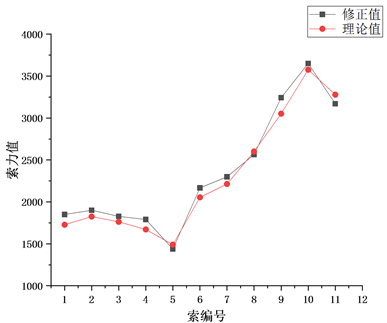Figure 1. Comparison of modified cable force and theoretical cable force

3. 油压回归法测换算索力

3.1. 建立回归方程

$F=\frac{P-0.4}{c}$ (6)

F：钢索拉力(kN)

P：油压表读数(MPa)

c：经验系数Table 2. Cable frequency and conversion cable force

3.2. 实际测量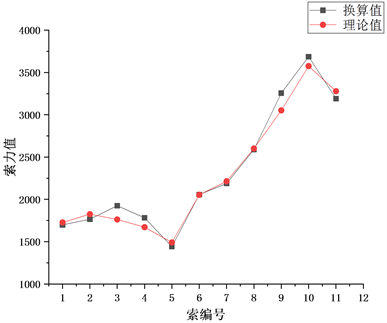Figure 2. Comparison of converted cable force and theoretical cable force

4. 等效法测等效索力

4.1. 理论基础

${E}_{eq}=\frac{{E}_{e}}{1+{E}_{e}\frac{A{q}^{2}{L}^{2}}{12{T}^{3}}}=\frac{{E}_{e}}{1+{E}_{e}\frac{{\gamma }^{2}{q}^{2}}{12{\sigma }^{3}}}$ (7)

Ee：斜拉索钢绞线弹性模量(MPa)；

γ：斜拉索容重(N/m3)；

L：斜拉索水平投影长度(m)， $L=l\cdot \mathrm{cos}\alpha$

σ：斜拉索索力(MPa)

$\Delta l=\underset{{T}_{0}}{\overset{{T}_{1}}{\int }}\frac{l}{{E}_{eq}A}dT=\left(\frac{{T}_{1}l}{EA}-\frac{{q}^{2}{l}^{3}{\mathrm{cos}}^{2}\alpha }{24{T}_{1}^{2}}\right)-\left(\frac{{T}_{0}l}{EA}-\frac{{q}^{2}{l}^{3}{\mathrm{cos}}^{2}\alpha }{24{T}_{0}^{2}}\right)$ (8)Table 3. Basic data of stay cables

4.2. 实际测量

5. 最优控制法控制最优索力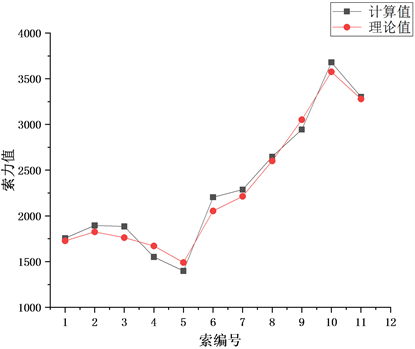Figure 3. Comparison of converted cable force and theoretical cable force

5.1. 构建最优控制模型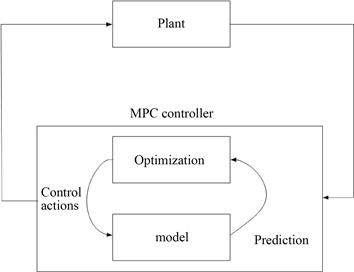Figure 4. Realization process of model predictive control

$\mathrm{min}J={\int }_{{t}_{0}}^{{t}_{f}}L\left(x\left(t\right),u\left(t\right),t\right)$ (9)

$x\left(t\right)$ ：状态变量

$u\left(t\right)$ ：控制变量

L：目标函数

5.2. 优化结果控制索力Table 4. Comparison of controlled cable force and theoretical cable force of stay cable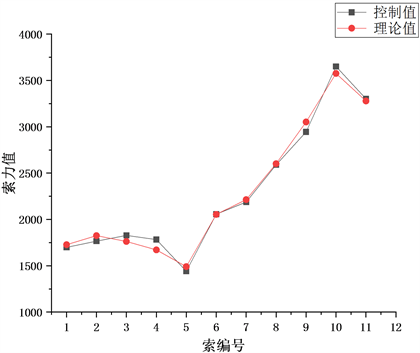Figure 5. Comparison of controlled cable force and theoretical cable force

6. 结语

1) 振动频率法将斜拉索振动近似作为弦的振动，通过明确弦的材料和长度后测量弦的振动频率确定弦的拉力。该方法便于对索力进行现场实时监测，其测量结果与理论索力值相差范围较为合理，可作为斜拉桥施工阶段索力实时监测的有效监测手段。

2) 油压回归法通过油压表读数建立回归方程求得千斤顶张拉力，即斜拉索张拉阶段的索力。作为振动频率法在拉索分级张拉阶段测量校核的重要依据，所得数据较为稳定，其测量数值也未超索力误差合理限值，在斜拉索张拉阶段可以在校核振动频率法所测索力的同时得到有效换算索力。

3) 等效法通过引入Ernst等效弹性模量，将斜拉索等效成一根弦杆，推导出索拉力和引伸量之间的函数关系，将索力等效转化为拉索引伸量，降低了索力测量难度的同时也提高了索力值精确度，因此该测量方法可作为梁段合拢后调索阶段索力的实际测量依据。

4) 针对三种测量方法中存在的局限，最优控制法将索力定义成为目标函数，将索力误差作为控制变量，把三种索力测量方法所得索力通过数学模型进行控制优化，提高了实测索力的精确度，减少了与理论索力之间的误差，实现了对实测索力值的优化。

 严国敏. 现代斜拉桥[M]. 成都: 西南交通大学出版社, 1989.

 林元培. 斜拉桥[M]. 北京: 人民交通出版社, 1994.

 Gimsing, S.J. (1997) Cable Supported Bridge. John Wiley, Chichester, 2-8.

 Podolmy, W. and Scalzi, J.B. (1976) Construction and Design of Cable-Stayed Bridge. John Wiley, New York.

 黄灿. 基于几何控制法的大跨度斜拉桥自适应施工控制体系研究[D]: [博士学位论文]. 成都: 西南交通大学, 2011.

 颜东煌, 刘光栋. 确定斜拉桥合理施工状态的正装迭代法[J]. 中国公路学报, 1999, 12(2): 61-66.

 秦顺全. 分阶段施工桥梁的无应力状态控制法[J]. 桥梁建设, 2008(1): 8-14.

 向中富, 徐君兰, 代正宏. 悬索桥施工控制分析的恒定无应力索长迭代法[J]. 重庆交通学院学报, 2000(3): 16-21.

 王晟, 宁平华, 颜东煌, 等. 基于索长迭代法的斜拉桥合理施工阶段索力研究[J]. 公路工程, 2019, 44(3): 6-10, 32.

 王晟, 颜东煌, 宁平华, 等. 确定钢桁梁斜拉桥合理施工阶段索力的索长迭代法[J]. 中外公路, 2019, 39(3): 78-82.

 李乔, 卜一之, 张清华. 基于几何控制的全过程自适应施工控制系统研究[J]. 土木工程学报, 2009, 42(7): 69-77.

Top